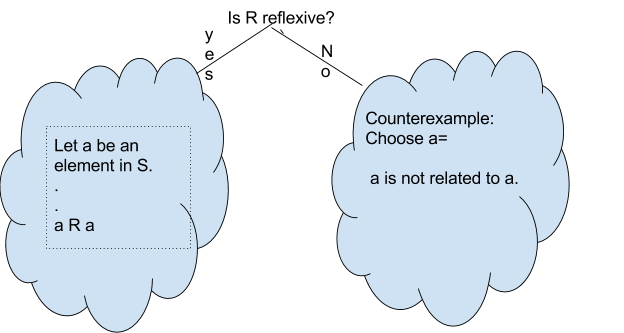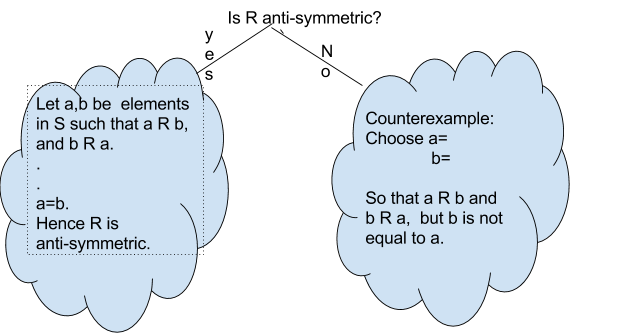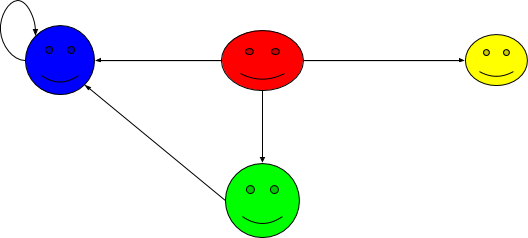$$\newcommand{\id}{\mathrm{id}}$$ $$\newcommand{\Span}{\mathrm{span}}$$ $$\newcommand{\kernel}{\mathrm{null}\,}$$ $$\newcommand{\range}{\mathrm{range}\,}$$ $$\newcommand{\RealPart}{\mathrm{Re}}$$ $$\newcommand{\ImaginaryPart}{\mathrm{Im}}$$ $$\newcommand{\Argument}{\mathrm{Arg}}$$ $$\newcommand{\norm}{\| #1 \|}$$ $$\newcommand{\inner}{\langle #1, #2 \rangle}$$ $$\newcommand{\Span}{\mathrm{span}}$$

# 2.1 Binary Relations

•• Contributed by Pamini Thangarajah
• Associate Professor (Mathematics & Computing) at Mount Royal University

$$\newcommand{\vecs}{\overset { \rightharpoonup} {\mathbf{#1}} }$$

$$\newcommand{\vecd}{\overset{-\!-\!\rightharpoonup}{\vphantom{a}\smash {#1}}}$$

Definition

Let $$S$$ be a non-empty set. Then any subset $$R$$ of $$S \times S$$ is said to be a relation over $$S$$. In other words, a relation is a rule that is defined between two elements in $$S$$. Intuitively, if $$R$$ is a relation over $$S$$, then the statement $$a R b$$ is either true or false for all $$a,b\in S$$.

Example $$\PageIndex{1}$$:

Let $$S=\{1,2,3\}$$. Define $$R$$ by $$a R b$$ if and only if $$a < b$$, for $$a, b \in S$$.

Then $$1 R 2, 1 R 3, 2 R 3$$ and $$2 \not R 1$$.

We can visualize the above binary relation as a graph, where the vertices are the elements of S, and there is an edge from $$a$$ to $$b$$ if and only if $$a R b$$ , for $$a,b\in S$$.The following are some examples of relation defined on $$\mathbb{Z}$$.

Example $$\PageIndex{2}$$:

1. Define $$R$$ by $$a R b$$ if and only if $$a < b$$, for $$a, b \in \mathbb{Z}$$.
2. Define $$R$$ by $$a R b$$ if and only if $$a >b$$, for $$a, b \in \mathbb{Z}$$.
3. Define $$R$$ by $$a R b$$ if and only if $$a \leq b$$, for $$a, b \in \mathbb{Z}$$.
4. Define $$R$$ by $$a R b$$ if and only if $$a \geq b$$, for $$a, b \in \mathbb{Z}$$.
5. Define $$R$$ by $$a R b$$ if and only if $$a = b$$, for $$a, b \in \mathbb{Z}$$.

Next, we will introduce the notion of "divides".

Definition

Let $$a$$ and $$b$$ be integers. We say that $$a$$ divides $$b$$ is denoted $$a\mid b$$, provided we have an integer $$m$$ such that $$b=am$$. In this case we can also say the following:

• $$b$$ is divisible by $$a$$
• $$a$$ is a factor of $$b$$
• $$a$$ is a divisor of $$b$$
• $$b$$ is a multiple of $$a$$

Example $$\PageIndex{3}$$:

$$4 \mid 12$$ and $$12 \not\mid 4$$

Theorem $$\PageIndex{1}$$: Divisibility inequality theorem

If $$a\mid b$$, for $$a, b \in \mathbb{Z_+}$$ then $$a \leq b$$,

Proof

Let $$a, b \in \mathbb{Z_+}$$ such that $$a\mid b$$ , Since (a\mid b\), there is a positive integer $$m$$ such that $$b=am$$. Since $$m \geq 1$$ and $$a$$ is a positive integer, $$b=am \geq (a)(1)=a.$$

Note that if $$a\mid b$$, for $$a, b \in \mathbb{Z_+}$$ then $$a \leq b$$, but the converse is not true. For example: $$2 <3$$, but $$2 \not\mid 3$$.

Example $$\PageIndex{4}$$:

According to our definition $$0 \mid 0$$.

Definition

An integer is even provided that it is divisible by $$2$$.

## Properties of binary relation:

Definition

Let $$S$$ be a set and $$R$$ be a binary relation on $$S$$. Then $$R$$ is said to be reflexive if $$a R a, \forall a \in S.$$

Example $$\PageIndex{5}$$: Visually$$\forall a \in S, a R a$$ holds.

We will follow the template below to answer the question about reflexive.Example $$\PageIndex{6}$$:

Define $$R$$ by $$a R b$$ if and only if $$a < b$$, for $$a, b \in \mathbb{Z}$$. Is $$R$$ reflexive?

Counter Example:

Choose $$a=2.$$

Since $$2 \not< 2$$, $$R$$ is not reflexive.

Example $$\PageIndex{7}$$:

Define $$R$$ by $$a R b$$ if and only if $$a \mid b$$, for $$a, b \in \mathbb{Z}$$. Is $$R$$ reflexive?

Proof:

Let $$a \in \mathbb{Z}$$. Since $$a=(1) (a)$$, $$a \mid a$$.

Thus $$R$$ is reflexive. $$\Box$$

Definition

Let $$S$$ be a set and $$R$$ be a binary relation on $$S$$. Then $$R$$ is said to be symmetric if the following statement is true:

$$\forall a,b \in S$$, if $$a R b$$ then $$b R a$$, in other words, $$\forall a,b \in S, a R b \implies b R a.$$

Example $$\PageIndex{8}$$: Visually$$\forall a,b \in S, a R b \implies b R a.$$ holds!

We will follow the template below to answer the question about symmetric.Example $$\PageIndex{7}$$:

Define $$R$$ by $$a R b$$ if and only if $$a < b$$, for $$a, b \in \mathbb{Z}$$. Is $$R$$ symmetric?

Counter Example:

$$1<2$$ but $$2 \not < 1$$.

Example $$\PageIndex{8}$$:

Define $$R$$ by $$a R b$$ if and only if $$a \mid b$$, for $$a, b \in \mathbb{Z}$$. Is $$R$$ symmetric?

Counter Example:

$$2 \mid 4$$ but $$4 \not \mid 2$$.

Definition

Let $$S$$ be a set and $$R$$ be a binary relation on $$S$$. Then $$R$$ is said to be antisymmetric if the following statement is true:

$$\forall a,b \in S$$, if $$a R b$$ and $$b R a$$, then $$a=b$$.

In other words, $$\forall a,b \in S$$, $$a R b \wedge b R a \implies a=b.$$

Example $$\PageIndex{9}$$: VISUALLY$$\forall a,b \in S$$, $$a R b \wedge b R a \implies a=b$$ holds!

We will follow the template below to answer the question about anti-symmetric.Example $$\PageIndex{10}$$:

Define $$R$$ by $$a R b$$ if and only if $$a < b$$, for $$a, b \in \mathbb{Z}$$. Is $$R$$ antisymmetric?

Example $$\PageIndex{11}$$:

Define $$R$$ by $$a R b$$ if and only if $$a \mid b$$, for $$a, b \in \mathbb{Z_+}$$. Is $$R$$ antisymmetric?

Definition

Let $$S$$ be a set and $$R$$ be a binary relation on $$S$$. Then $$R$$ is said to be transitive if the following statement is true

$$\forall a,b,c \in S,$$ if $$a R b$$ and $$b R c$$, then $$a R c$$ .

In other words, $$\forall a,b,c \in S$$, $$a R b \wedge b R c \implies a R c$$.

Example $$\PageIndex{12}$$: VISUALLY$$\forall a,b,c \in S$$, $$a R b \wedge b R c \implies a R c$$ holds!

We will follow the template below to answer the question about transitive.Example $$\PageIndex{13}$$:

Define $$R$$ by $$a R b$$ if and only if $$a < b$$, for $$a, b \in \mathbb{Z}$$. Is $$R$$ transitive?

Example $$\PageIndex{14}$$:

Define $$R$$ by $$a R b$$ if and only if $$a \mid b$$, for $$a, b \in \mathbb{Z_+}$$. Is $$R$$ transitive?

Summary:

In this section we learned about binary relation and the following properties:

Reflexive

Symmetric

Antisymmetric

Transitive